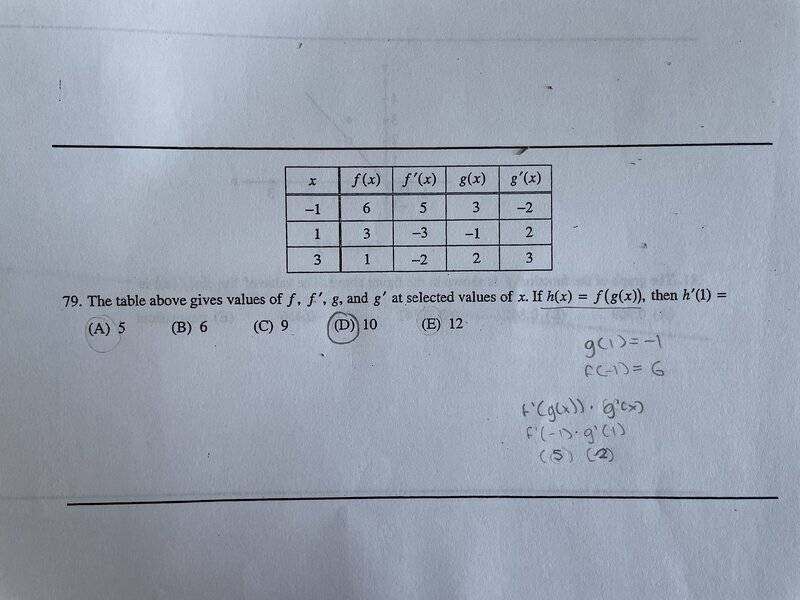# Derivatives and the chain rule

• AL107

#### AL107

Homework Statement
x: -1 1 3
f(x): 6 3. 1
f’(x): 5. -3 -2
g(x): 3. -1. 2
g’(x): -2. 2. 3

The table above gives values of f, f', g, and g' at selected values of x. If h(x) = f(g(x)), then h'(1) =
(A) 5
(B) 6
(C) 9
(D) 10
(E) 12
Relevant Equations
h(x)=f(g(x))
I originally thought you’d have to use the chain rule to get h’, as in: f’(g(x))*g’(x). Plugging in 1 for x, I got an answer of 10. An online solution, however, said that you only had to get f(g(1)), which was f(-1), then look up f’(-1) in the table. Both approaches seem logical to me, but they yield different results. Can someone clarify? Thank you!

Oh, here is a better table:An online solution, however, said that you only had to get f(g(1)), which was f(-1), then look up f’(-1) in the table.
How can that possibly be right? The chain rule applies.

•AL107
How can that possibly be right? The chain rule applies.
Thank you!

In the second method, you are differentiating h by x and f by g, so you are not performing the same operation on both sides of the equation. Be careful when using the prime notation: h'(x) means dh/dx, but f'(g) means df/dg. It may be helpful to write out the derivatives explicitly:
h(x) = f(g)
dh/dx = df/dx = df/dg*dg/dx

•AL107
Intuitively, you can not just look at f' because x has to go through g before f is applied. Consider the simple example, f(x)=x. Then h(x)=f(g(x)) = g(x) and clearly h'= g', so g' can not be ignored.

•AL107